# Some improved criteria on exponential stability of neutral differential equation

## Abstract

In this paper, some improved delay-dependent exponential stability criteria of the neutral delayed differential equation

$d d t [ x ( t ) + p x ( t − τ ( t ) ) ] +ax(t)−btanhx ( t − σ ( t ) ) =0,$

are expressed in terms of linear matrix inequalities (LMIs). Compared with some existing ones, our results are derived without the use of the mode transformation method and the bounding technique. Three numerical examples are provided to show the effectiveness of the proposed method and less conservatism of the obtained results.

## 1 Introduction

In this paper, we reconsider the following neutral delayed differential equation:

$d d t [ x ( t ) + p x ( t − τ ( t ) ) ] +ax(t)−btanhx ( t − σ ( t ) ) =0,$
(1)

for $t≥0$, where a, b are two positive real constants and $|p|<1$. The delays $τ(⋅):[0,+∞)→[0,τ]$ ($τ>0$) and $σ(⋅):[0,+∞)→[0,σ]$ ($σ>0$) are bounded functions and $r=max{τ,σ}>0$. There exist two positive constants $μ 1 , μ 2 ∈(0,1)$ such that $τ ′ (t)≤ μ 1$, $σ ′ (t)≤ μ 2$. For each solution of equation (1), we assume the initial condition

$x 0 (θ)=ϕ(θ),θ∈[−r,0],$

where $ϕ∈C([−r,0];R)$.

When $τ(t)≡τ$ and $σ(t)≡σ$, the system (1) is turned into the following form:

$d d t [ x ( t ) + p x ( t − τ ) ] +ax(t)−btanhx(t−σ)=0,t≥0.$
(2)

Recently, for equation (2), many authors have investigated its properties due to its applications in the research of the dynamic characteristics of neural networks of Hopfield type, see  and references therein. Although some qualitative stability analysis for equation (2) have been presented in [1, 3, 514, 17], their results are mainly concerned with the asymptotic stability, without providing any conditions for exponential stability and any information about the decay rates of equation (2). Besides, it should be pointed out that the advantage of equation (2) with exponential stability in comparison with that with asymptotic stability lies in that the former can provide fast convergence and desirable accuracy. In , Li has obtained the global exponential stability for equation (2), but the sufficient condition is delay-independent. It is well known that current efforts on the problem of stability for delay systems can be divided into two categories, namely delay-independent stability criteria and delay-dependent stability criteria. And generally speaking, the former are more conservative than the latter when the delay is small. Thus, the sufficient condition about the global exponential stability for equation (2) given in  is more conservative. More recently, in , Rojsiraphisal and Niamsup have proposed some delay-dependent LMI-based sufficient conditions ensuring the exponential stability for equation (2), but these conditions are also more conservative since the mode transformation and the bounding technique are both used. On the other hand, to the best of our knowledge, compared with constant-delay systems, the time-varying delay systems are more closed to reflect the reality. So, it is necessary to discuss the global exponential stability for equation (1). In , Chen and Meng have established the LMI-based exponential stability criterion for equation (1) by only constructing a modified Lyapunov functional, but the result in  is sightly more conservative. Thus, there still exists room for further improvement.

In this paper, we reconsider the exponential stability of equation (1). New delay-dependent sufficient criteria ensuring the global exponential stability for equation (1) are given in terms of linear matrix inequalities (LMIs). The criteria here are also discussed from the point of view of its comparison with the earlier results. To show the applicability and effectiveness of the proposed method developed in this paper, three illustrative examples are provided.

Notation Throughout this paper, the notation $X≥Y$ (respectively, $X>Y$) means that the matrix $X−Y$ is positive semi-definite (respectively, positive definite), where X and Y are symmetric matrices of the same dimensions. The symbol denotes the elements below the main diagonal of a symmetric matrix.

## 2 Main results

In this section, we give our main results.

Theorem 1 For a given positive constant $κ>0$, the zero solution of equation (1) is globally exponentially stable if there exist some positive scalars: $α 0$, $α 1$, $α 2$, $α 3$ and $α 4$, such that the following linear matrix inequalities (LMIs) hold:

$Ω 1 = [ Ω 11 1 Ω 12 1 λ 1 α 0 b 0 ∗ Ω 22 1 λ 1 α 0 p b 0 ∗ ∗ − λ 1 α 3 ( 1 − μ 2 ) 0 ∗ ∗ ∗ − α 2 τ ] < 0 , Ω 2 = [ Ω 11 2 Ω 12 2 λ 2 α 0 b 0 ∗ Ω 22 2 λ 2 α 0 p b 0 ∗ ∗ − α 3 λ 2 ( 1 − μ 2 ) 0 ∗ ∗ ∗ − α 4 σ ] < 0 ,$
(3)

where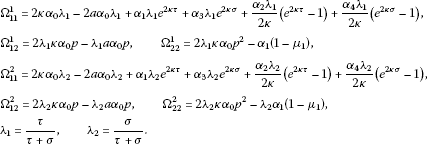Proof Consider the Lyapunov functional defined by

$V ( t , x ( t ) ) = e 2 κ t α 0 [ x ( t ) + p x ( t − τ ( t ) ) ] 2 + α 1 ∫ t − τ ( t ) t e 2 κ ( s + τ ) x 2 ( s ) d s + α 2 ∫ − τ 0 ∫ t + θ t e 2 κ ( s − θ ) x 2 ( s ) d s d θ + α 3 ∫ t − σ ( t ) t e 2 κ ( s + σ ) tanh 2 x ( s ) d s + α 4 ∫ − σ 0 ∫ t + θ t e 2 κ ( s − θ ) tanh 2 x ( s ) d s d θ ,$
(4)

where $α i$ ($i=0,1,2,3,4$) are positive scalars to be chosen later.

The derivative of $V(t,x(t))$ along the solution of equation (1) is determined by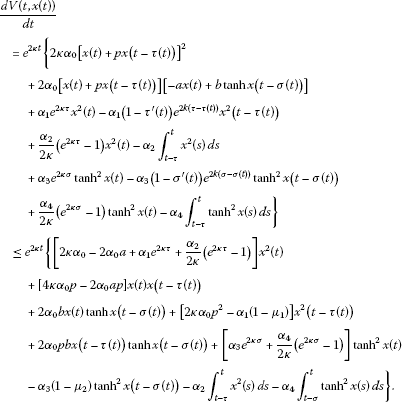(5)

The fact that $tanh 2 x(t)≤ x 2 (t)$ implies

$[ α 3 e 2 κ σ + α 4 2 κ ( e 2 κ σ − 1 ) ] tanh 2 x(t)≤ [ α 3 e 2 κ σ + α 4 2 κ ( e 2 κ σ − 1 ) ] x 2 (t).$
(6)

Substituting (6) into (5), we have

$d V ( t , x ( t ) ) d t ≤ 1 τ ∫ t − τ t ξ 1 T (t,s) Ω 1 ξ 1 (t,s)ds+ 1 σ ∫ t − σ t ξ 2 T (t,s) Ω 2 ξ 2 (t,s)ds,$
(7)

where $ξ 1 (t,s)= [ x T ( t ) , x T ( t − τ ( t ) ) , tanh T x ( t − σ ( t ) ) , x T ( s ) ] T$ and $ξ 2 (t,s)= [ x T ( t ) , x T ( t − τ ( t ) ) , tanh T x ( t − σ ( t ) ) , tanh T x ( s ) ] T$.

From (3), we have $d V ( t , x ( t ) ) d t <0$, which implies $V(t,x(t))≤V(0,x(0))$. And from the definition of the Lypunov function $V(t,x(t))$, we have

$V ( 0 , x ( 0 ) ) = α 0 [ x ( 0 ) + p x ( − τ ( 0 ) ) ] 2 + α 1 ∫ − τ ( 0 ) 0 e 2 κ ( s + τ ) x 2 ( s ) d s + α 2 ∫ − τ 0 ∫ θ 0 e 2 κ ( s − θ ) x 2 ( s ) d s d θ + α 3 ∫ − σ ( 0 ) 0 e 2 κ ( s + σ ) tanh 2 x ( s ) d s + α 4 ∫ − σ 0 ∫ θ 0 e 2 κ ( s − θ ) tanh 2 x ( θ ) d θ d s ≤ [ 4 α 0 + ( α 1 + α 3 ) r e 2 κ r + ( α 2 + α 4 ) e 2 κ r 4 κ 2 ] sup θ ∈ [ − r , 0 ] | ϕ ( θ ) | 2 ≡ M .$

So, we obtain

$| x ( t ) + p x ( t − τ ( t ) ) | 2 ≤ M 1 e − 2 κ t ,$
(8)

where $M 1 = M α 0 >0$. For $∀ε∈(0,min{2κ,− 2 r log|p|})$ and $ν>0$, the fundamental inequality $xy≤ν x 2 + 1 ν y 2$ for any $x,y∈R$ implies

$e ε t | x ( t ) | 2 ≤ ( 1 + ν ) e ε t | x ( t ) + p x ( t − τ ( t ) ) | 2 + 1 + ν ν e ε t | p x ( t − τ ( t ) ) | 2 ≤ ( 1 + ν ) M 1 + 1 + ν ν | p | 2 e ε r e ε ( t − τ ( t ) ) | x ( t − τ ( t ) ) | 2 .$

And from $ε∈(0,min{2κ,− 2 r log|p|})$, we have $| p | 2 e ε r <1$. Thus, as $ν>0$ is chosen sufficiently large,

$ϵ= | p | 2 ( 1 + ν ) e ε r ν <1.$

Therefore, we have

$e ε t | x ( t ) | 2 ≤(1+ν) M 1 +ϵ e ε ( t − τ ( t ) ) | x ( t − τ ( t ) ) | 2 .$
(9)

About (9), for $∀T≥0$,

$sup 0 ≤ t ≤ T { e ε t | x ( t ) | 2 } ≤(1+ν) M 1 +ϵ sup θ ∈ [ − r , 0 ] | ϕ ( θ ) | 2 +ϵ sup 0 ≤ t ≤ T { e ε t | x ( t ) | 2 } .$

Consequently,

$sup 0 ≤ t ≤ T { e ε t | x ( t ) | 2 } ≤ ( 1 + ν ) M 1 + ϵ sup θ ∈ [ − r , 0 ] | ϕ ( θ ) | 2 1 − ϵ .$
(10)

As $T→+∞$, it follows from (10) that

$sup 0 ≤ t < + ∞ [ e ε t | x ( t ) | 2 ] ≤ ( 1 + ν ) M 1 + ϵ sup θ ∈ [ − r , 0 ] | ϕ ( θ ) | 2 1 − ϵ ,$

that is,

$| x ( t ) | ≤ M 2 e − α t ,$

where $M 2 = ( 1 + ν ) M 1 + ϵ sup θ ∈ [ − r , 0 ] | ϕ ( θ ) | 2 1 − ϵ >0$ and $α= ε 2 >0$. The proof of this theorem is completed. □

When $τ(t)≡τ,σ(t)≡σ$, we can easily derive the following corollary.

Corollary 2 For a given positive constant $κ>0$, the zero solution of equation (2) is globally exponentially stable if there exist some positive scalars: $α 0$, $α 1$, $α 2$, $α 3$, $α 4$, such that the following linear matrix inequalities (LMIs) hold: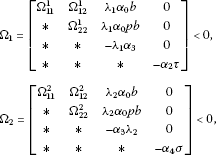where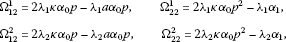and $Ω 11 1$, $Ω 11 2$, $λ 1$, $λ 2$ are given in Theorem 1.

Remark 1 The proofs of Theorem 1 and Corollary 2 are completed by utilizing the technique involved in , so that the mode-transformation technique and the bounding technique are not employed. Although one LMI-based sufficient condition ensuring the exponential stability for equation (2) has been obtained in , this condition is more conservative since the mode-transformation technique and the bounding technique are both used, and the obtained result has narrow applications since the condition

$|p|+(σ−τ)|b|<1,$
(11)

must be imposed. Besides, note that Theorem 4 in  involves seven decision variables, while Corollary 2 involves five decision variables. Thus, Corollary 2 needs fewer decision variables than Theorem 4 in . What is more, this restrictive condition (11) is removed in this paper. Thus, our LMIs-based sufficient conditions are less conservative than those provided in , which is shown by Example 1 and Example 2 in Section 3. And the technique employed in this paper is different from the previous ones introduced in [1, 3, 711, 16, 17].

Remark 2 Although the delay-independent sufficient condition for the global exponential stability of equation (2) has been obtained, the technique used in  is only suitable for constant delay, not for time-varying delays. So, our result can complement the result in . Besides, in , the delay-independent sufficient condition for the global exponential stability of equation (2) has been given in the form

$a ( 1 − | p | ) >b ( 1 + | p | ) .$
(12)

Remark 3 If $κ=0$, the criteria about the global asymptotical stability for equation (1) are presented as follows:

Corollary 3 The zero solution of equation (1) is globally asymptotically stable if there exist some positive scalars: $α 0$, $α 1$, $α 2$, $α 3$ and $α 4$, such that the following linear matrix inequalities (LMIs) hold:

$Ω 1 = [ Ω 11 1 Ω 12 1 λ 1 α 0 b 0 ∗ Ω 22 1 λ 1 α 0 p b 0 ∗ ∗ − λ 1 α 3 ( 1 − μ 2 ) 0 ∗ ∗ ∗ − α 2 τ ] < 0 , Ω 2 = [ Ω 11 2 Ω 12 2 λ 2 α 0 b 0 ∗ Ω 22 2 λ 2 α 0 p b 0 ∗ ∗ − λ 2 α 3 ( 1 − μ 2 ) 0 ∗ ∗ ∗ − α 4 σ ] < 0 ,$
(13)

$where$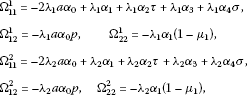$λ i$ ($i=1,2$) are given in Theorem  1.

## 3 Three illustrative examples

In this section, three illustrative examples are given to show the effectiveness of our result.

Example 1 Consider the following equation in [3, 7, 911, 16, 18]:

$d d t [ x ( t ) + 0.2 x ( t − 0.1 ) ] =−0.6x(t)+0.3tanhx(t−σ).$
(14)

By Corollary 2, if $κ=0.0038$ is given, the upper bound of the time-delay σ which guarantees the exponential stability of equation (14) is 175.289. Obviously, when $σ=175.289$, the two inequalities (11) and (12) do not hold and both criteria given in [8, 16] have a narrow application. And, by Corollary 3, the upper bound of time-varying delay $σ(t)$ for the global asymptotic stability of equation (14) is $1.38× 10 21$. The comparison results of the maximum allowable delay bounds σ in [3, 7, 911, 16, 18] and our result are listed in Table 1. Obviously, our result is less conservative than those in [3, 7, 911, 16, 18]. By solving the LMIs (13) in Corollary 3, with respect to $α 0$, $α 1$, $α 2$, $α 3$, $α 4$, we obtain a solution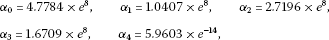when the maximum value of σ is $1.38× 10 21$.

Example 2 Consider the following equation in [1, 3, 5, 710, 16, 18]:

$d d t [ x ( t ) + 0.35 x ( t − 0.5 ) ] =−1.5x(t)+btanhx(t−0.5).$
(15)

By Corollary 2, if $κ=0.177$ is given, we obtain that the upper bound of b about the global exponential stability of equation (15) is 1.0929. And by Corollary 3, the upper bound of b ensuring the global asymptotic stability of equation (15) is up to 1.405. Obviously, it is seen from Table 2 that our result is better than those given in [1, 3, 5, 710, 16, 18]. By solving the LMIs (13) with respect to $α 0$, $α 1$, $α 2$, $α 3$, $α 4$, if $κ=0.177$, we obtain a solutionwhen the maximum value of b is 1.0929. When $b=1.0929$, the criterion about the global exponential stability of equation (15) is not obtained in  since the inequality (12) is not satisfied. It is easily seen that our result is less conservative than one in . The simulation for the trajectory of the solution to such a system when $σ=τ=0.5$ and $b=1.405$ is given in Figure 1.

Example 3 Consider the following equation with time-varying delays :

$d d t [ x ( t ) + 0.2 x ( t − τ ( t ) ) ] =−0.6x(t)+0.5tanhx ( t − σ ( t ) ) ,$
(16)

where $τ(t)= sin 2 ( t ) 10$ and $μ 2 =0.2$. By using Corollary 3, the upper bound of time-varying delay $σ(t)$ for asymptotic stability of equation (16) is $1.285× 10 25$. But, by virtue of Theorem 1 in , the maximin allowable bound of time-varying delay $σ(t)$ for asymptotic stability of this equation is $5.000× 10 24$. So, Corollary 3 is better than one in . When $σ=1.285× 10 25$, by the Matlab LMIs Control Toolbox, a solution to the LMIs (13) in Corollary 3 can be obtained as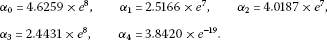Obviously, the result provided in  is infeasible. And even if the delays $τ(t)$ and $σ(t)$ are the constant delays, the zero solution of equation (16) is not globally exponentially stable according to the criterion given in  since the inequality (12) is not satisfied. Thus, our result can also complement that in [8, 16].

## References

1. 1.

Agarwal RP, Grace SR: Asymptotic stability of certain neutral differential equations. Math. Comput. Model. 2000, 31: 9–15.

2. 2.

Rakkiyappan R, Balasubramaniam P: New global exponential stability results for neutral type neural networks with distributed time delays. Neurocomputing 2008, 71: 1039–1045. 10.1016/j.neucom.2007.11.002

3. 3.

Deng S, Liao X, Guo S: Asymptotic stability analysis of certain neutral differential equations: a descriptor system approach. Math. Comput. Simul. 2009, 71: 4297–4308.

4. 4.

Fridman E: New Lyapunov-Krasovskii functionals for stability of linear retarded and neutral type systems. Syst. Control Lett. 2001, 43: 309–319. 10.1016/S0167-6911(01)00114-1

5. 5.

EI-Morshedy HA, Gopalsamy K: Nonoscillation, oscillation and convergence of a class of neutral equations. Nonlinear Anal. 2000, 40: 173–183. 10.1016/S0362-546X(00)85010-5

6. 6.

Kulenovic M, Ladas G, Meimaridou A: Necessary and sufficient conditions for oscillations of neutral differential equations. J. Aust. Math. Soc. Ser. B, Appl. Math 1987, 28: 362–375. 10.1017/S0334270000005452

7. 7.

Kwon OM, Park J: On improved delay-dependent stability criterion of certain neutral differential equations. Appl. Math. Comput. 2008, 199: 385–391. 10.1016/j.amc.2007.09.031

8. 8.

Li X: Global exponential stability for a class of neural networks. Appl. Math. Lett. 2009, 22: 1235–1239. 10.1016/j.aml.2009.01.036

9. 9.

Nam P, Phat V: An improved stability criterion for a class of neutral differential equations. Appl. Math. Lett. 2009, 22: 31–35. 10.1016/j.aml.2007.11.006

10. 10.

Park JH, Kwon OM: Stability analysis of certain nonlinear differential equations. Chaos Solitons Fractals 2008, 37: 450–453. 10.1016/j.chaos.2006.09.015

11. 11.

Park JH: Delay-dependent criterion for asymptotic stability of a class of neutral equations. Appl. Math. Lett. 2004, 17: 1203–1206. 10.1016/j.aml.2003.05.013

12. 12.

Park JH, Kwon O: On new stability criterion for delay-differential systems of neutral type. Appl. Math. Comput. 2005, 162: 627–637. 10.1016/j.amc.2004.01.001

13. 13.

Park JH, Jung HY: On the exponential stability of a class of nonlinear systems including delayed perturbations. J. Comput. Appl. Math. 2003, 159: 467–471. 10.1016/S0377-0427(03)00550-8

14. 14.

Park JH, Won S: Stability analysis for neutral delay-differential systems. J. Franklin Inst. 2000, 337: 1–9. 10.1016/S0016-0032(99)00040-X

15. 15.

Lien C, Yu K, Lin Y, Chung Y, Chung L: Global exponential stability for uncertain delayed neural networks of neutral type with mixed time delays. IEEE Trans. Syst. Man Cybern., Part B, Cybern. 2008, 38: 709–720.

16. 16.

Rojsiraphisal T, Niamsup P: Exponential stability of certain neutral differential equations. Appl. Math. Comput. 2010, 217(8):3875–3880. 10.1016/j.amc.2010.09.047

17. 17.

Sun Y, Wang L: Note on asymptotic stability of a class of neutral differential equations. Appl. Math. Lett. 2006, 19: 949–953. 10.1016/j.aml.2005.10.015

18. 18.

Chen H, Meng X: An improved exponential stability criteria for a class of neutral delayed differential equations. Appl. Math. Lett. 2011, 24: 1763–1767. 10.1016/j.aml.2010.10.007

19. 19.

Xu S, Lam J: Improved delay-dependent stability criteria for time-delay systems. IEEE Trans. Autom. Control 2005, 50: 384–387.

## Acknowledgements

The author would like to thank the anonymous referees for their very helpful comments and suggestions which greatly improved this manuscript, and the editors for their careful reading of this paper. This work is supported by the National Natural Science Foundation of China under Grant No. 11126278 and the Natural Science Foundation of Jiangxi Province in China under Grant No. 20114BAB211001.

## Author information

Authors

### Corresponding author

Correspondence to Huabin Chen.

### Competing interests

The author declares that he has no competing interests.

### Authors’ contributions

The author has solely completed the whole works in this paper.

## Authors’ original submitted files for images

Below are the links to the authors’ original submitted files for images.

## Rights and permissions

Reprints and Permissions

Chen, H. Some improved criteria on exponential stability of neutral differential equation. Adv Differ Equ 2012, 170 (2012). https://doi.org/10.1186/1687-1847-2012-170